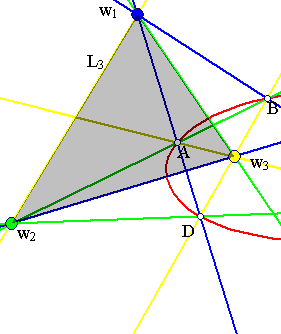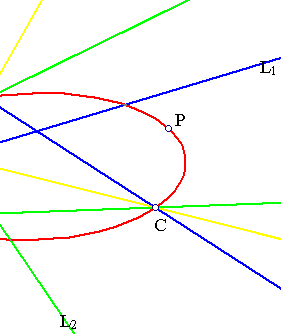##Family of conics

A family of conics I(a,b) is defined by two conics. If the conics are described by the equations f(x,y) = 0, g(x,y) = 0, then the family consists of conics described by the equation hs,t(x,y) = 0, where
hs,t(x,y) = sf(x,y) + tg(x,y).

Consider the representation of the conics through symmetric matrices, so that conics (a), (b) are represented respectively through equations of the form xtA1x = 0 and xtA2x = 0. Then the family generated by the two conics (a) and (b) has members represented by equations of the form:
xtAs,tx = 0, where the symmetric matrix As,t = sA1 + tA2.

A crucial role in the discussion plays the determinant d(s,t) = |As,t| and in particular its roots, which determine the singular (or degenerate) conic-members of the family I(a,b). Assuming A1, A2 to be non-singular, obviously there are at most three. They are obtained by solving the homogeneous equation d(s,t) = 0, or equivalently solving the eigenvalue problem:
|sA1 + tA2| = 0 <==> |A1A2-1 + t/s| = 0.

In the generic case, in which A1A2-1 has three different real eigenvalues, the corresponding three eigenvectors v1, v2, v3 represent three points of the projective plane, satisfying the eigenvector equation:
A1A2-1vi + (ti/si)vi = 0, and setting wi = A2-1vi ==> siA1wi + tiA2wi = 0.
Since (ytAw = 0, variable y) represents the polar line of w with respect to A, the previous equation implies that wi have the same polar line Li with respect to all members of the family.The above figure shows a typical family consisting of all conics passing through four points {A,B,C,D} in general position. The family is generated by any two members (degenerate or not) and contains three degenerate conics represented by the pairs of opposite sides of the complete quadrilateral ABCD.
w1, w2, w3 coincide with the intersection points of the opposite sides and the polar Li of wi contains the other two wj,s. This can be seen algebraically by using the equations: siA1wi + tiA2wi = 0, sjA1wj + tjA2wj = 0, multiplying the first by wj, the second by wi substracting etc..
The triangle w1w2w3 is self-polar (or autopolar) with respect to any member of the family I(a,b).
Another use of wi's is frequently made by considering the images F(L) of lines under the so-called quadratic transformation F, defined by the family. These images F(L) are namely conics passing through the points w1, w2, w3.

### See Also

Quadratic_Transformation.html
Quadratic_Transformation2.html

### References

Berger, M Geometry II Paris, Springer Verlag, 1987, Chapter 16.5 Pencils of conics, p. 192.
Berger Marcel, Pansu Pierre, Berry Jean-Pic, Saint-Raymond Xavier Problems in Geometry Paris, Springer Verlag 1984, p. 98.

 Produced with EucliDraw© http://users.math.uoc.gr/~pamfilos/